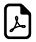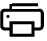Eight Times Tables - Column Trick8 Times Multiplication Table TricksSteps to Learn Eight Times Table Easy

STEP1:In column-1, write 8, 7, 6, 5, 4, 4, 3, 2, 1, 0

STEP2:In column-2, write 0, 2, 4, 6, 8, 0, 2, 4, 6, 8

STEP3:In column-3, Merge the column-1 & Column-2, See the results in below table.

Column-1 Column-2 Merge Column-1 & 2x8 Times Table
8 0 80 10 x 8 = 80
7 2 72 9 x 8 = 72
6 4 64 8 x 8 = 64
5 6 56 7 x 8 = 56
4 8 48 6 x 8 = 48
4 0 40 5 x 8 = 40
3 2 32 4 x 8 = 32
2 4 24 3 x 8 = 24
1 6 16 2 x 8 = 16
0 8 8 1 x 8 = 8

Think 8 times Table.Think Reverse !!!How 8 times table works?

Put a table with 4 column and 10 rows.

Column1: write in reverse order like 8, 7, 6, 5, 4, 4, 3, 2, 1, 0

Column2: write even numbers till 8 like 0, 2, 4, 6, 8, 0, 2, 4, 6, 8

Column3: Merge Column1 and Column2Times Table Tricks 2 to 11►

2 Times Table Trick

4 Times Table Trick

5 Times Table Trick

6 & 7 Times Table Trick

8 Times Table TrickTop Calculators ►

Tax Calculator, Calories Burned Calculator, PERT, SD Calculator, Dog Age Calculator, Children Equal Playing Time Calculator